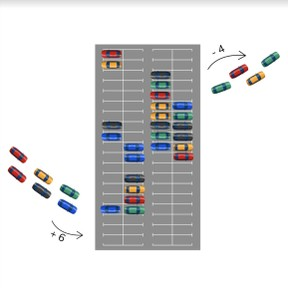Word Problems with addition & subtraction to 100

# Word Problems with addition & subtraction to 100

Use addition and subtraction in word problems up to 100.

No account needed.8,000 schools use Gynzy92,000 teachers use Gynzy1,600,000 students use Gynzy

## General

Students learn to add and subtract up to 100 in the context of story problems.

## Standards

CCSS.Math.Content.2.OA.A.1

## Learning objective

Students will be able to add and subtract in word problems with numbers up to 20.

## Introduction

Practice addition and subtraction problems to 100. Ask six students to come to the front of the class and divide them into two groups. Give them an addition or subtraction problem with numbers to 100 and the group that has the correct answer first gets a point. You can also choose to play this as a class or with individual students.

## Instruction

Start by walking students through the steps of solving a story problem, reminding them that they are looking for the numbers they need to solve the math problem. In the first step students look at the story and determine what kind of math problem it is. How do you recognize that something will be added? How do you recognize that something will be subtracted? In the second step students determine which numbers are important to the math problem. In the third step students say or write the math problem, and the fourth step is to solve the math problem. Discuss each step individually with examples. Then practice the steps consecutively with the students with a problem. Then ask students to solve a story problem individually or in pairs without showing them the steps. Have students explain their strategy and talk through the steps they take to solve the story problem.

To check that students are able to use addition and subtraction to 100 in story problems you can ask the following questions:
- How do you know that you need to add in a story problem?
- How do you know that you need to subtract in a story problem?
- Why is it useful to say or write the math problem before solving the math problem?

## Quiz

Students are initially given word problems in a story form with visual support. They then progress to stories without visual support. Finally students are also given problems in which they must add and subtract, or add/subtract multiple times within the same problem.

## Closing

Check that students are able to solve story problems by asking them about how to solve story problems. To close the lesson ask students to solve the three story problems individually. Then you can decide either to have students discuss their answers in groups, or to ask a student to explain their strategy to the class.

## Teaching tips

Students who have difficulty solving these problems individually, can be helped by walking through the steps together. Start with simple problems, for example: Marco swims 14 laps, and Lia swims 23 laps. How many laps did they swim together? Walk through the steps together and as they gain confidence, make the addition or subtraction problems more challenging.

### The online teaching platform for interactive whiteboards and displays in schools

• Save time building lessons

• Manage the classroom more efficiently

• Increase student engagement